# Weber problem

(diff) ← Older revision | Latest revision (diff) | Newer revision → (diff)

A problem formulated in [a7] as a model for the optimum location of a facility in the plane intended to serve several users; for example, a central source of electric power. One is seeking the minimum of a function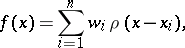where the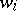are positive scalars, the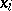are given vectors in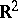,is inandis the Euclidean norm of. The case when all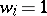andhad been considered by P. Fermat in 1629, by E. Torricelli in 1644 and by J. Steiner in 1837. (For the early history of the problem, see [a4].)

The functionis convex (cf. Convex function (of a real variable)) and one shows that, with some exceptions, it has a unique minimizer. These assertions remain valid whenis allowed to be an arbitrary norm andis replaced by.

For applications, of which there are many (see [a1]), one seeks good computational methods for finding a minimizer of, either with the Euclidean norm or with other norms. For the Euclidean case, E. Weiszfeld [a8] provided a much used method; see [a6] for a discussion of this and other cases. Ifis the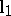-norm, explicit solution is possible (see [a2], Chap. 4).

Minimizing the functionis a problem in optimization and it is natural to seek a dual problem (cf. Duality in extremal problems and convex analysis): to maximize a functionsuch that. A dual was found for special cases by H.W. Kuhn and others [a4]. A major result in this direction was provided by C. Witzgall in [a9], who provided a dual for a more general minimum problem, in which the function to be minimized has the form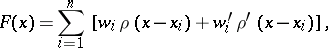whereis now allowed to be an asymmetric norm in(that is,is required to be valid only for non-negative) and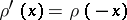. Witzgall's result and others are subsumed under a duality theorem of W. Kaplan and W.H. Yang [a3]. In this theorem, the functionhas the formin, whereis a norm, allowed to be asymmetric, in,is a constant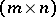-matrix,in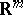andinare constant vectors. The dual functionis the linear functionin, subject to the constraintsand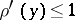, whereandare dual norms in: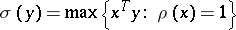. It is assumed that the equation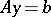has a solution with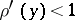. In [a3] it is shown how, when the norms are differentiable (except at the origin), a minimizer ofcan be obtained from or determines a maximizer of. It is also shown that the theorem provides a dual for the multi-facility location problem, for which the functionto be minimized is the sum of the weighted distances fromnew facilities togiven facilities as well as the weighted distances between the new facilities; the functionis a convex function of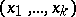, where theth new facility is placed at.

The Weber problem has been generalized in many ways to fit the great variety of problems arising in the location of facilities. See [a1] for an overview.

How to Cite This Entry:
Weber problem. Encyclopedia of Mathematics. URL: http://encyclopediaofmath.org/index.php?title=Weber_problem&oldid=16979
This article was adapted from an original article by Wilfred Kaplan (originator), which appeared in Encyclopedia of Mathematics - ISBN 1402006098. See original article